# Ratio Summary - Line item & time.Hello Team,

I was wondering that can we have different ratio for line items and time. For ratio as summary in Anaplan we have only one option, which will calculate the same for both line items and Time.

Any suggestions, can we have different summaries by any work around.

Thanks,
Manjunath

Tagged:

•I am not sure about the use case to which you are trying to apply this solution.

But I could achieve this using two additional line items (Numerator and Denominator) in which you will calculate the expected values based on condition which checks if it is time summary or non-time summary. This will be a little confusing, so please bear with me.

1. This is the data which I will be using as numerator and denominator of the ratio summary based on Time and Non-Time (Other) dimension.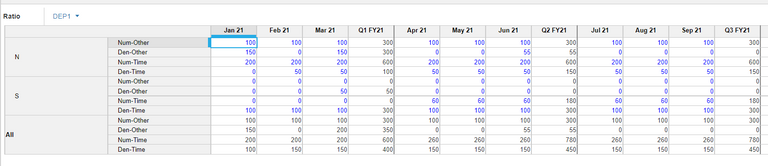2. The logic to determine Time and Non-time dimension is as follows: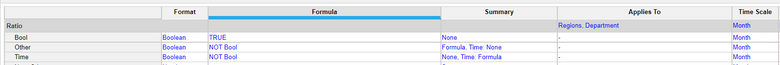This is how it looks like in the module - Time checked in the time summaries and Other checked in Non-time summaries: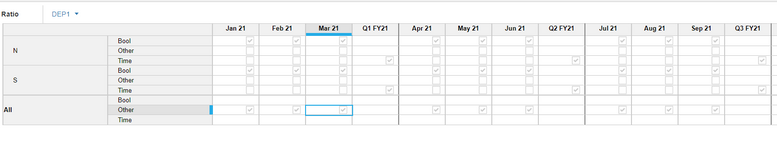3. Then create the Numerator and Denominator line items with Formula summary which are calculated based on the above booleans.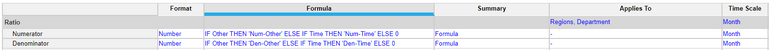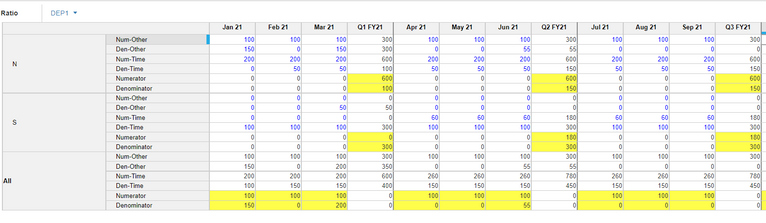4. Set the Numerator and Denominator line items in the Ratio summary.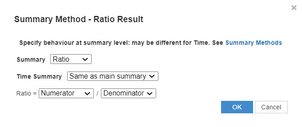The highlighted values show the ratio summary calculated.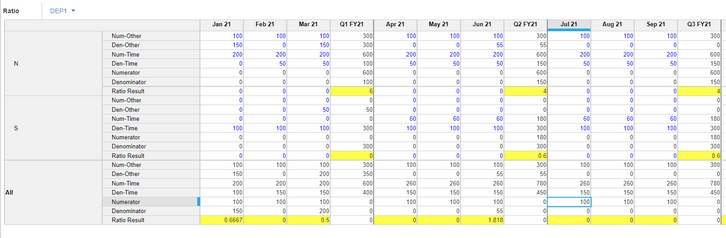Let me know if this works for your question. Thanks.

•This is nice, I am sorry if I am not able to convey properly. Based on your example. I am asking you my requirement. I want something like.

numerator 1 and denominator 1 in time summary of ratio result, numerator 2 & denominator 2 in line item summary of ratio result. All 4 are different line items,

Thanks,

Manjunath

•If I understood it right, the solution achieves the same thing.

In the above solution:

numerator 1 and denominator 1 in time summary = Num-Time and Den-Time

numerator 2 and denominator 2 in line item summary = Num-Other and Den-Other

All four being different line items to be used for the ratio summary.

•Hi @shreyashree,

Really appreciate the work but I wanted the result in Line items in all list members, not just the top level. In your answer, Line item ratio result is having "0" values in list members, I understand you are overwriting the summary by different line items.

Thanks,
Manjunath

•Hi @ManjunathKN ,
We could achieve that by putting in N/D as the formula for that line item right? Or could you maybe provide an example of the ask here to better understand what is required?
Thanks!